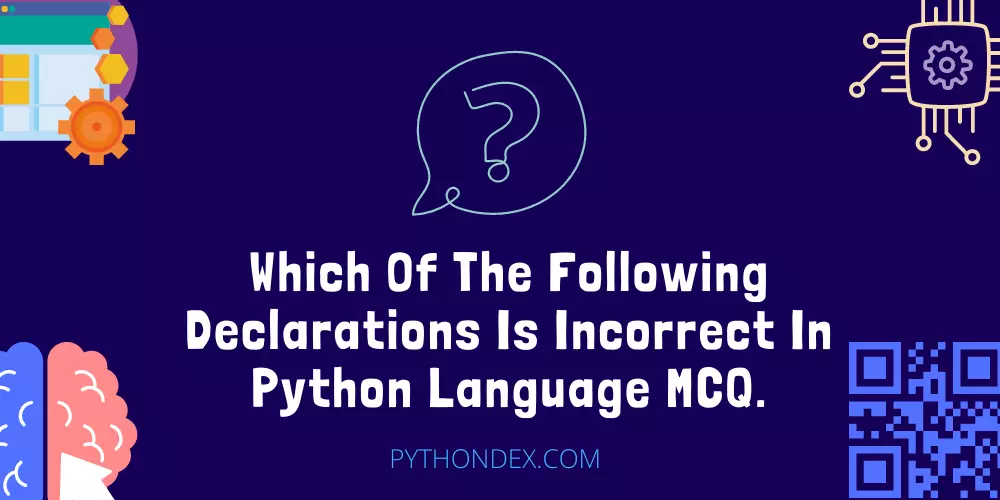ExplorePython MCQ'S# Which Of The Following Declarations Is Incorrect In Python Language MCQ.Want to test your python programming knowledge, then you are at the right place in this article. You can test your python knowledge by answering which of the following declarations is incorrect in python language MCQ.

Which of the following declarations is incorrect in python language MCQ is a type of MCQ where you will have to tell an incorrect python declaration from the given choices.

This python MCQ will help you to improve your python knowledge and make you a better python programmer. If you want another python MCQ you can go here: What will be the output of following python code MCQ.

The Complete Python Programming Boo...
The Complete Python Programming Bootcamp

You will get a question and multiple choices you need to think and decide which is the correct declaration then you can check your answer by clicking the view answer button so now let’s start.

You can test the declaration below in this online python compiler.

## 1. Which of the following declaration is incorrect in python language

1. print(‘Hello world’)
2. print(``Hello world``)
3. print(“Hello world”)
4. All of the above

## 2. Which of the following declaration is incorrect in python language

1. nums = 100 200 300 400
2. nums = 100,200,300,400
3. nums = 100200300400
4. All of the above

## 3. Which of the following declaration is incorrect in python language

1. print(“Very Good’)
2. print(“`Hello world`“)
3. print(‘Hello world’)
4. All of the above

## 4. Which of the following declaration is incorrect in python language

1. if (n < 1): // code
2. if n > 1: // code
3. if (n > 1) { // code }
4. All of the above

## 5. Which of the following declaration is incorrect in python language

1. print(‘Hello world’)
2. print(``Hello world``)
3. print(“Hello world”)
4. All of the above

## 6. Which of the following declaration is incorrect in python language

1. num_1 = 1
2. num = 1
3. 1num = 1
4. All of the above

## 7. Which of the following declaration is incorrect in python language

1. my-name = ‘stark’
2. my_name = ‘stark’
3. myname = ‘stark’
4. All of the above

## 8. Which of the following declaration is correct for declaring function in python language

1. def hello: //code
2. def hello(num): //code
3. def hello(): //code
4. All of the above

## 9. Which of the following declaration is correct for declaring list in python language

1. nums = [1,2,3,4,5]
2. nums = (1,2,3,4,5)
3. nums = 1,2,3,4,5
4. All of the above

## 10. Which of the following declaration is incorrect in python language

1. import [itex]
2. import math
3. import “math”
4. All of the above